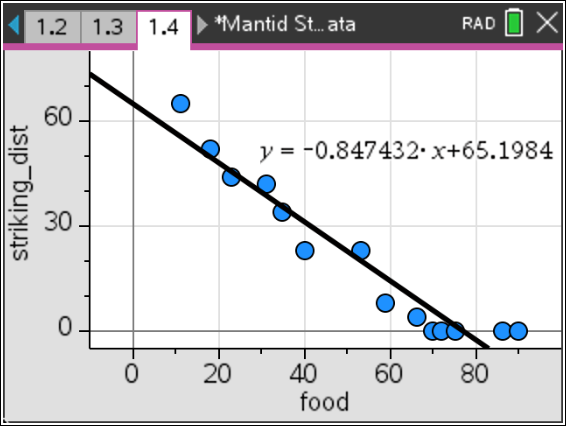# Activities

•• ##### Subject Area

• Math: Mathematical Modeling: Prediction

6-8
9-12

50 Minutes

• ##### Device
•TI-Nspire™ CX/CX II
•TI-Nspire™ CX CAS/CX II CAS
• ##### Software

TI-Nspire™ CX
TI-Nspire™ CX CAS

5.0

## Modeling Mantid Striking Distance#### Activity Overview

In this TI-Nspire activity, students investigate the relationship between how hungry a praying mantis (also called a “mantid”) is and the distance at which the praying mantis will strike (jump) at prey.

#### Objectives

Students will be able to:

• Use functions to model relationships between quantities.
• Summarize, represent, and interpret data on two quantitative variables.
• Describe qualitatively the functional relationship between two quantities by analyzing a graph.
• Fit a function to data and use functions fitted to data to solve problems in the context of the data.

#### About the Lesson

This activity is appropriate for students with basic knowledge of linear and quadratic functions typically covered in an introductory algebra course. It is not necessary for students to have had experience with linear regression, but the activity affords a natural opportunity to consider regression models.

Modeling is often associated with fitting a formula to data in a way that can be used for prediction. Scatter plots are used to display paired data, and regression is a mathematical tool for finding a function that best fits the plot. However, data come from a contextual situation, and simply “goodness of fit” is not the only measure of the appropriateness of a model.